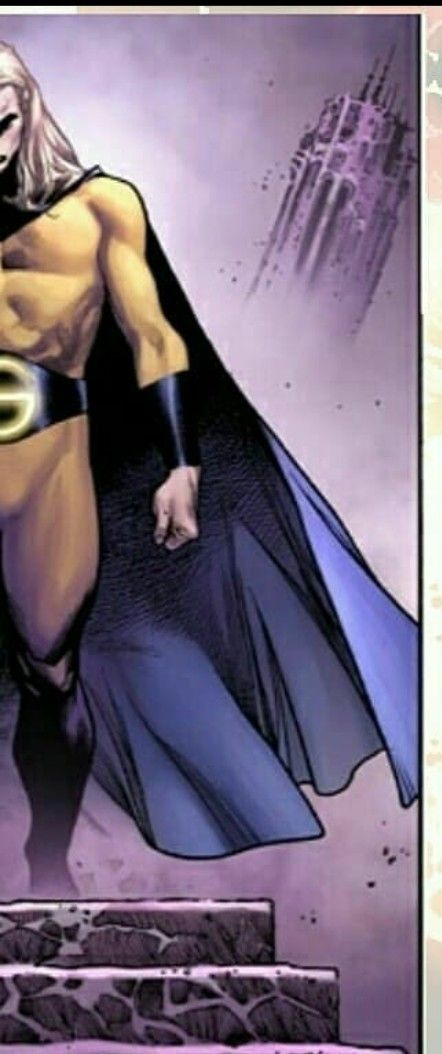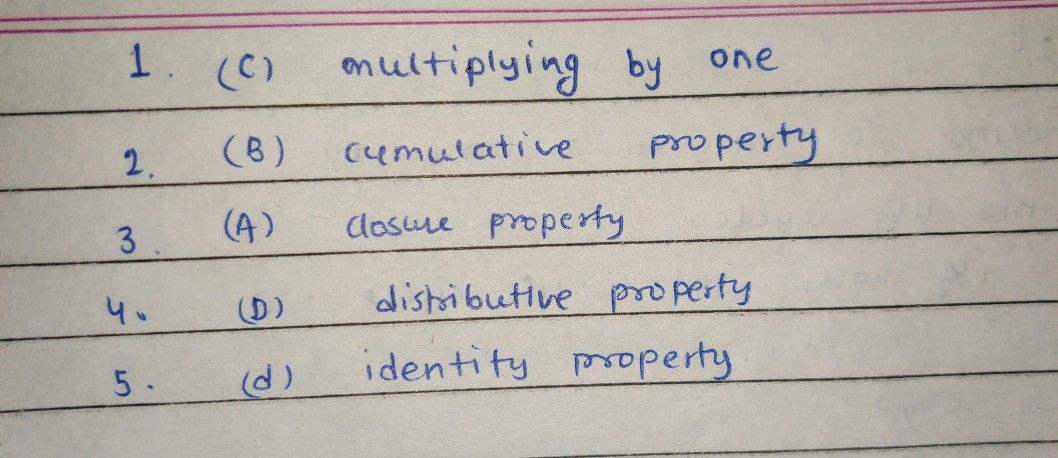Symbol
Problemer of integers by answering in ts Choose the letter of the best answer. Write the chosen letter on a separate sheet of paper 1. Which operation below does not change $1bc$ value of any nonzero number? A Adding by One B. Dividing by Zero C. Multiplying by One D. Multiplying by Zero 2. Which of the following property states that order $oftw0$ $angethe$ mucltihpalniegd indg otes he not change numbers that are either being added or value, $A$ $Clos11r$ property B. Commutative property C. Associative property D. Identity property $3$ Which of the following property states that the $y0$ $integer$ that $ar0$ added and multiplied remain as integers? The set of integers is closed under addition and multiplication $A$ Closure property B. Commutative property C. Associative property D. Distributive property $4$ When two numbers have been added / subtracted and then $nultiplied$ $loya$ factor, the result will be the same when each number is multiplied by the factor and the products are then added / subtracted. A. Closure property $B$ $Comm11tativ$ property C. Associative property D. Distributive property $5$ Which of the following property states that the sum of any number $and0is$ the given number? A. Closure property $B.$ Commutative property $C.1$ Associative property D. Identity property
7th-9th grade
Probability and Statistics
Search count: 111
SolutionQanda teacher - Aryanhi dear feel free to ask queries please evaluate the answer if satisfied
Please give 3000 coins as thank you gift this will not reduce your coins
happy Learning ?❣️?